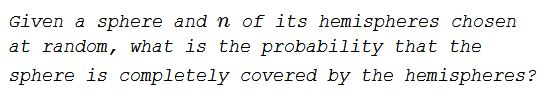# Hemisphere Coverage

### Problem### Solution

For a start, let's observe that some configurations, like when, for example, three or more of the hemisphere bounding great circles concur, may not fall in line with general reasoning. But such configurations occur with probability $0$ and may be ignored.

Each hemisphere is completely bounded by a great circle. The $n$ great circles divide the surface of the sphere into $n^2-n+2$ regions (which can be proved by induction.) Each great circle bounds two hemispheres. Hence, the probability that a given point, not on the great circle, is in one of the hemispheres is $2^{-1}.$ The probability that it is covered by none of the $n$ hemispheres is $2^{-n}.$ The probability that the point lies on one of the great circles is zero. Other than that, a point belongs to exactly one of the $n^2-n+2$ regions. If the point is not covered by the hemispheres, so is the region it belongs to. Thus, the probability that at least one of those regions is not covered by the hemispheres is $2^{-n}(n^2-n+2).$ The probability that the entire sphere is covered is then $1-2^{-n}(n^2-n+2).$

For $n=1,$ the formula gives the probability of $0,$ which is obviously true because a single hemisphere only covers half a sphere, not the whole of it. For $n=2,$ the probability is also $0,$ although two hemispheres, if bounded by the same great circle, cover the entire sphere. The probability of this is the same as that of having a random point on the sphere fall on a given great circle, which is $0.$ For $n=3,$ the formula again gives zero probability.

### Acknowledgment

The problem and the solution are from Beautiful Mathematics, by Martin Erickson.

I am grateful to Vincent Pantaloni who pointed to a slip in reasoning in an earlier version of the page.

A 2D variant is available elsewhere.